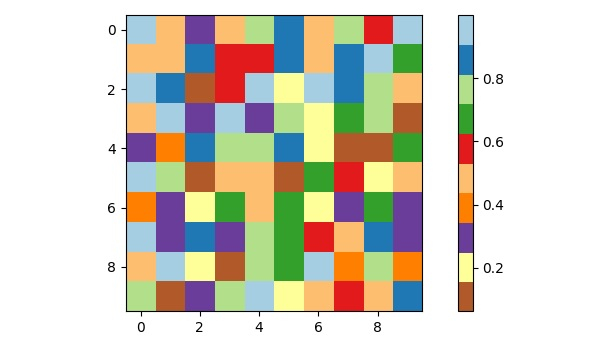# Defining a discrete colormap for imshow in Matplotlib

To define a discrete colormap for imshow in matplotlib, we can take following the steps −

• Create data using numpy.

• Initialize the data using get_cmap, so that scatter knows.

• Using imshow() method with colormap, display the data as an image, i.e., on a 2D regular raster.

• Create the colorbar using the colorbar() method.

• To display the figure, use the show() method.

## Example

import numpy as np
from matplotlib import pyplot as plt
import matplotlib
plt.rcParams["figure.figsize"] = [7.50, 3.50]
plt.rcParams["figure.autolayout"] = True
data = np.random.rand(10, 10)
cmap = matplotlib.cm.get_cmap('Paired_r', 10)
plt.imshow(data, cmap=cmap)
plt.colorbar()
plt.show()

## OutputUpdated on: 09-Apr-2021

1K+ Views